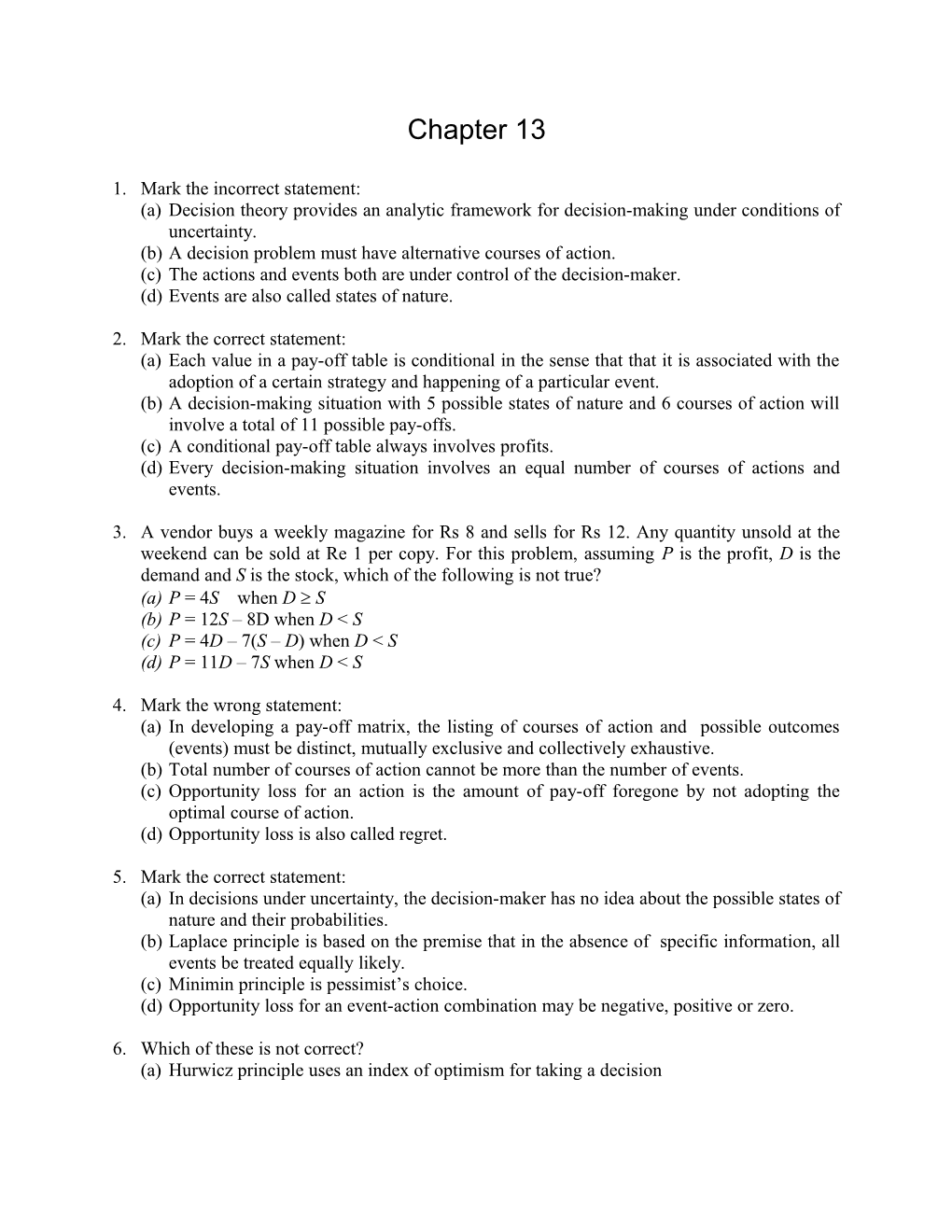# (A)Decision Theory Provides an Analytic Framework for Decision-Making Under ConditionsChapter 13

1. Mark the incorrect statement:

(a)Decision theory provides an analytic framework for decision-making under conditions of uncertainty.

(b)A decision problem must have alternative courses of action.

(c)The actions and events both are under control of the decision-maker.

(d)Events are also called states of nature.

1. Mark the correct statement:

(a)Each value in a pay-off table is conditional in the sense that that it is associated with the adoption of a certain strategy and happening of a particular event.

(b)A decision-making situation with 5 possible states of nature and 6 courses of action will involve a total of 11 possible pay-offs.

(c)A conditional pay-off table always involves profits.

(d)Every decision-making situation involves an equal number of courses of actions and events.

1. A vendor buys a weekly magazine for Rs 8 and sells for Rs 12. Any quantity unsold at the weekend can be sold at Re 1 per copy. For this problem, assuming P is the profit, D is the demand and S is the stock, which of the following is not true?

(a)P = 4S when DS

(b)P = 12S – 8D when DS

(c)P = 4D – 7(S – D) when DS

(d)P = 11D – 7S when DS

1. Mark the wrong statement:

(a)In developing a pay-off matrix, the listing of courses of action and possible outcomes (events) must be distinct, mutually exclusive and collectively exhaustive.

(b)Total number of courses of action cannot be more than the number of events.

(c)Opportunity loss for an action is the amount of pay-off foregone by not adopting the optimal course of action.

(d)Opportunity loss is also called regret.

1. Mark the correct statement:

(a)In decisions under uncertainty, the decision-maker has no idea about the possible states of nature and their probabilities.

(b)Laplace principle is based on the premise that in the absence of specific information, all events be treated equally likely.

(c)Minimin principle is pessimist’s choice.

(d)Opportunity loss for an event-action combination may be negative, positive or zero.

1. Which of these is not correct?

(a)Hurwicz principle uses an index of optimism for taking a decision

(b)For a given strategy in a profit maximising problem, Hurwicz criterion =  Maximum profit + (1 - )  Minimum profit.

(c)For a profit maximising decision problem, the minimax principle involves choosing minimum of the maximum pay-offs, since a pessimist adopts such a policy.

(d)Savage principle uses the concept of regret.

1. Mark the correct statement:

(a)Under expectation principle, the expected pay-offs for each of the states of nature are calculated for taking decision.

(b)For a given problem, the decisions under expected cost principle and expected regret principle would be different.

(c)Decision on expected regret basis underlies the Savage principle.

(d)For any strategy, the expected regret value refers to the summation of the products of regret values and the corresponding probabilities.

1. Choose the wrong statement:

(a)EPPI is the summation of the products of the payoff corresponding to optimal policy in every event and the probability of the event.

(b)EVPI cannot exceed EPPI.

(c)ECPI is the expected cost under perfect information.

(d)EPPI is equal to the expected regret corresponding to optimal course of action.

1. Mark the wrong statement:

(a)In Bayesian approach, the optimal strategy is chosen on the basis of expected value criterion using posterior probabilities.

(b)Posterior probabilities are calculated from prior probabilities with the help of conditional and joint probabilities.

(c)Posterior probabilities are obtained as ratio of joint probabilities and total probability.

(d)The sum of prior probabilities, conditional probabilities and posterior probabilities has each to be equal to one.

1. Mark the correct statement:

(a)EVSI is expected value of strategic information.

(b)Efficiency of EVSI = (EVSI / EPPI)  100

(c)EVSI is the difference between expected payoffs with and without sample information.

(d)Higher the value of EVSI in relation to EVPI, lower the efficiency of sample information.

1. Choose the wrong statement about decision-trees:

(a)They are used where sequential decision-making is involved.

(b)This approach uses expectation principle for decision-making.

(c)The decision is reached by rollback technique.

(d)The rollback technique involves rolling the tree from left to right.

1. Mark the wrong statement: :

(a)As a basis of decision-making, utility approach uses expected utility.

(b)Utility function provides a scale for converting monetary values into utils.

(c)Utility approach to decision-making is objective in nature.

(d)Utility function must possess the property of completeness.

1. Mark the wrong statement:

(a)A util is an imaginary unit.

(b)The utility function of a risk-neutral decision-maker is linear.

(c)A risk-seeker has increasing marginal utility of money.

(d)For a given problem, the decision under EMV and EU criteria would always be identical.

1. Mark the incorrect statement:

(a)If U(0) = 0 and U(10) = 5, then a bet with outcomes of zero and 10 with probabilities 0.3 and 0.7 respectively has expected utility equal to 3.5.

(b)A utility function always ranges between zero and 100.

(c)The utility approach to decision-making assumes that if an individual indifferent between two alternatives A1 and A2, then A1 and A2 have equal utility for him.

(d)Von Neumann and Morgenstern provided the concept of index for measurement of utility.

1. Mark the correct statement:

(a)Maximum likelihood and expectation principles deal with decision-making under risk.

(b)Expected regret criterion leads to same decision as the expected pay-off criterion in a given situation.

(c)The probabilities of various outcomes at each chance node of a decision tree may or may not add to 1.

(d)A utility function holds for a particular individual in a given situation only.